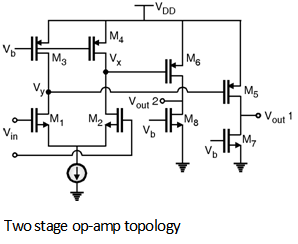Home > Analog CMOS Design > CMOS Opamp > Two Stage Op amp

Two Stage Op-amp :

In some applications the gain provided by single stage op-amps is not sufficient. In such circuits two stage op-amps are used. The first state of the op-amp provides the required large gain and second stage gives the large voltage swings. Figure below shows the simple two state op-amp circuit.The first stage of the op-amp is a differential pair consists of transistors m1, m2, m3 and m4. The output Va and Vy are the differential output 6 which are given by,

Vx = gm2 (r02 || r04) V in and

Vy = gm1 (r01 || r03) V in

These voltages Vx and Vy are then further amplified by the 2nd stage which a is consists of the transistors m 5, m7 and m6, m8. The output voltage at the 2nd stage is given by,

Vout1 = gm5 (r05 || r07) V y and

Vout2 = gm6 (r06 || r08) V x.

\ The total gain of the op-amp is the product of the gains of the stages which is given as,

Av = g m1 g m5 (r01 || r 03) (r05 || r07)

or It can be also given as,

Av = g m2 g m6 (r02 || r 04) (r06 || r08)